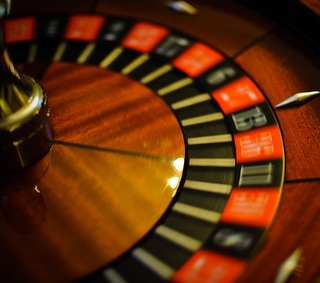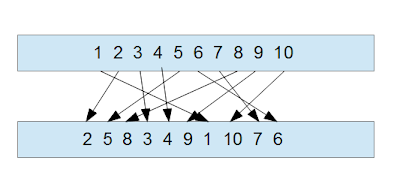### Optimization Horizons: system and algorithmic trading

New horizons of optimization

## Monte Carlo analysis for trading results for system risk control for real newbiesThis an article that I wrote about Monte Carlo analysis of trading results for system risk control.

I hope you will like it.

The Monte Carclo analysis is applied for the risk control of trading systems. It can aswer the question how much risk you can get with a given trading sytem. Beyound what level you need to drop the system.

"What is the last thing you do before you climb on a ladder? You shake it. And that is a Monte Carlo simulation"
Sam Savage, Stanford University

So if we make an analogy the ladder is the result of the trading system's back tests.

Have a look at the picture. Here we have 10 trades of our imaginary trading system, they are numbered from 1 to 10. Each trade is either good or bad, what is important is that we have an equity curve that is formed by those trades.

Here you can imagine we have a result consisting of 10 trades. What we do is that we shaken those results randomly, however no trade is added or deleted. What we do is that we just replace their position randomly. This is called selection without replacement. In other words we change the positions of the trade sequence of the system.By doing selection without replacement we duplicate the probability distribution of the initial trade sequence.

If we apply this many times on an initial trading sequence we will get something that looks like that, check the screen shot.

The beginning and the end is at the same point because it is the same trading sequence, but the paths are different.

However the next questions arise, how many permutations we can do? 10, 100, 10000 or 100000.

The practical limits are given by the statistics.

Imagine we have 1000 trades. By calculating the permutations that will give us:

1000*999*998*997*...3*2*1

The number we get is unbelievably big. The number of permutations of 200 is bigger than the number of all atoms that exist in the whole universe. So it if obvious we are not going to do all those permutations.
Hopefully there is a simple algorithm that will help us:

If the probability p is close to 0.5 So the 95 % confidence interval reduces to the following formula:

If we have a flipping coin of 1000 times and we want a confidence level of 95 %. The confidence level will explain how likely the 500 heads is placed within the confidence interval around the estimation.

For the Gaussian distribution we can be 95 % sure that the value is accurate within 3.1 %. With 95 % probability the coin will will fall between 469 and 531 times a head from the 1000 trials you can do.

If we use the formula we get

0.5+1/sqrt(1000)= 0.5+ 0.3162277

0.5-1/sqrt(1000)=0.5- 0.3162277

So we can share that the value is 95 % sure that is accurate within 3.1%.

Practical use of Monte Carlo simulation for traders

So what does it means in practice. In fact the most important aspect of this kind of Monte Carlo analysis is the analysis of the drawdowns. For example with a given confidence level you can say what that worse drowdown can be.

Look again at the shot where are the equity curves. We are going to be interested at the worse case scenario.

Imagine the original system has 500 USD drow down an 1500 USD average profit per year. In our hypothetic example that would be the system with the blue line.

So now we are going to look at the hypothetical equity line with the worst drow down.

If your Monte Carlo analysis show you for example 1000 USD as the worse drowdawn at 95 % confidence level that means that there is 5 % chance of facing 1000 USD drow downs before making any profits. Well if you look at even lower confidence levels the expected drowdown can be lower.

However the worst drow down is not more than the average profit of the system. According to Urban Jaeckle and Emilio Tomasini it is unacceptable to have a drowdown lower than the average profit per year.

The main limitation of the Monte Carlo analysis are that if your initial results are curve – fitted your results will be a nonsense. The Monte Carlo analysis is good only when it is applied for a sound trading system and not to an over-fitted one.

If your trading system is sound or not that is just another thing. The Monte Carlo simulation need to be applied with care but it is a valuable tool for risk control of a sound trading system.

Urban Jaeckle, Emilio Tomasini, 'Trading Systems A new approach to system development and portoflio optimization' Hariman house, p.101-108

Farell, Christopher, 'Monte Carlo models simulate all kinds of scenarios' Business Week 2001

Discrete Event Simulation a First course at this link

• stevegee58 3301 days ago

I hadn't considered this before.  I've seen many systems that were profitable or not depending on the starting point.  By mixing up the trade results you can shed more light on if a system is really good or bad.

• JohnLast 3301 days ago

I suggest you take a loot at the book Urban Jaeckle, Emilio Tomasini, 'Trading Systems A new approach to system development and portoflio optimization' Hariman house, p.101-108

It is quite interesting.

• JohnLast 3299 days ago

I checked what is available on FF regarding the use of Monte Carlo simulation. Here I found spread sheets for Monte Carlo simulation.
However I think not every system can be modeled using those spreadsheets

In those spreadsheets it is about modeling system expectancy. So it would be OK if your system has fixed stops and take profit levels so you can calculate exactly the win/loss. By doing that you can model it.

However if you use stop and reverse signals and no stop loss, or if you use a time filter or trailing stop. I think that in those case the system expectancy is not modeled properly.

• jaguar1637 3297 days ago

Yes, the Monte Carlo is one of the new topic.

I would like to have a look on Levenmark algorythm too

• JohnLast 3269 days ago

I was experimenting with excel sheets of Monte Carlo simulation but they were doing a diferent thing. Based on the performance of the system: % of profit trades and profit factor it simulates the hypothetic performance of the system. But this is something else and I wonder of it possible application for the evaluation of trading systems.

However the tool is interesting to see how the changes in the % of profit trades and profit factor affect the possible equity curves.

There are some paid excel addons doing selection without replacement monte carlo simulations. I even found a free video tutorials but it looks quite tricky for me and requires some coding for excel to get something usefull.